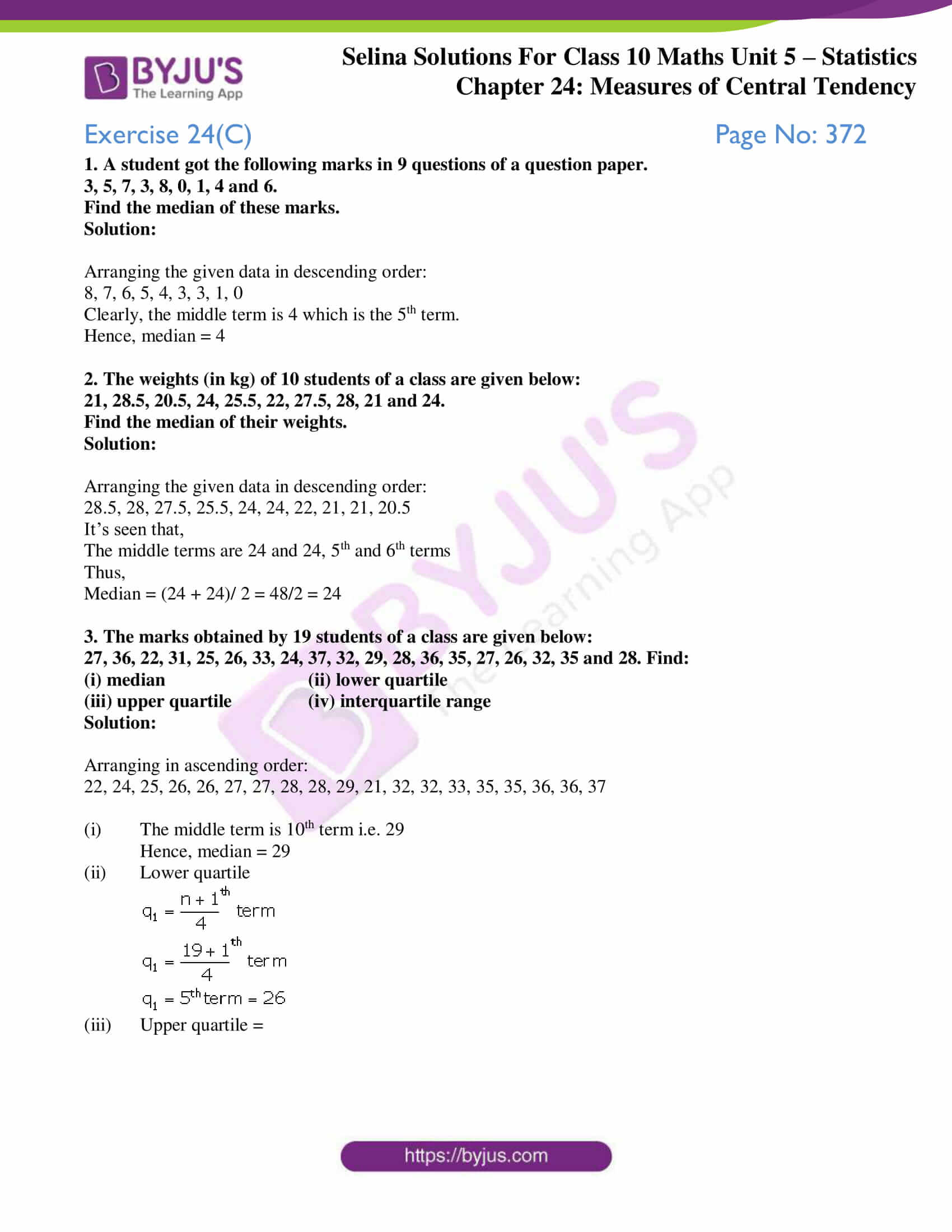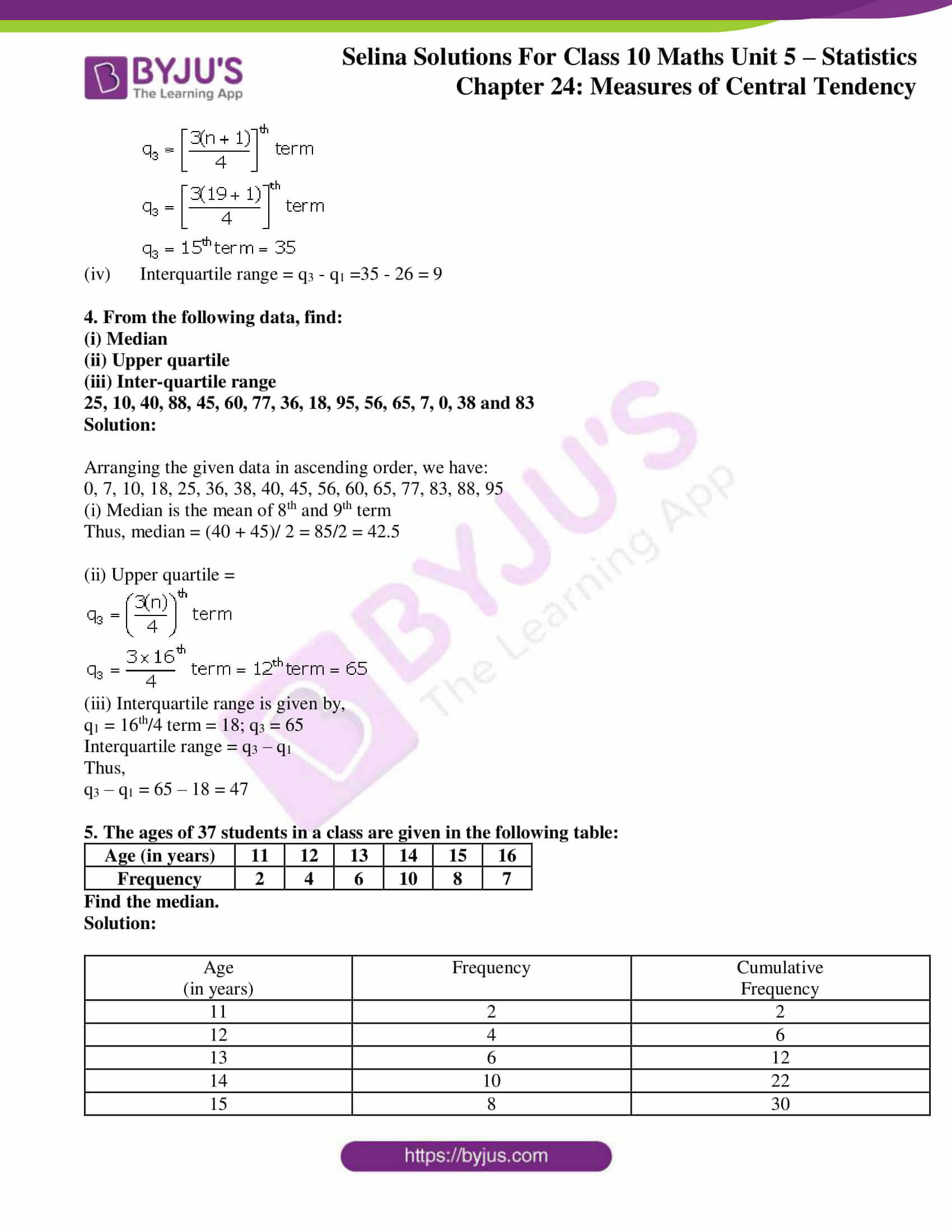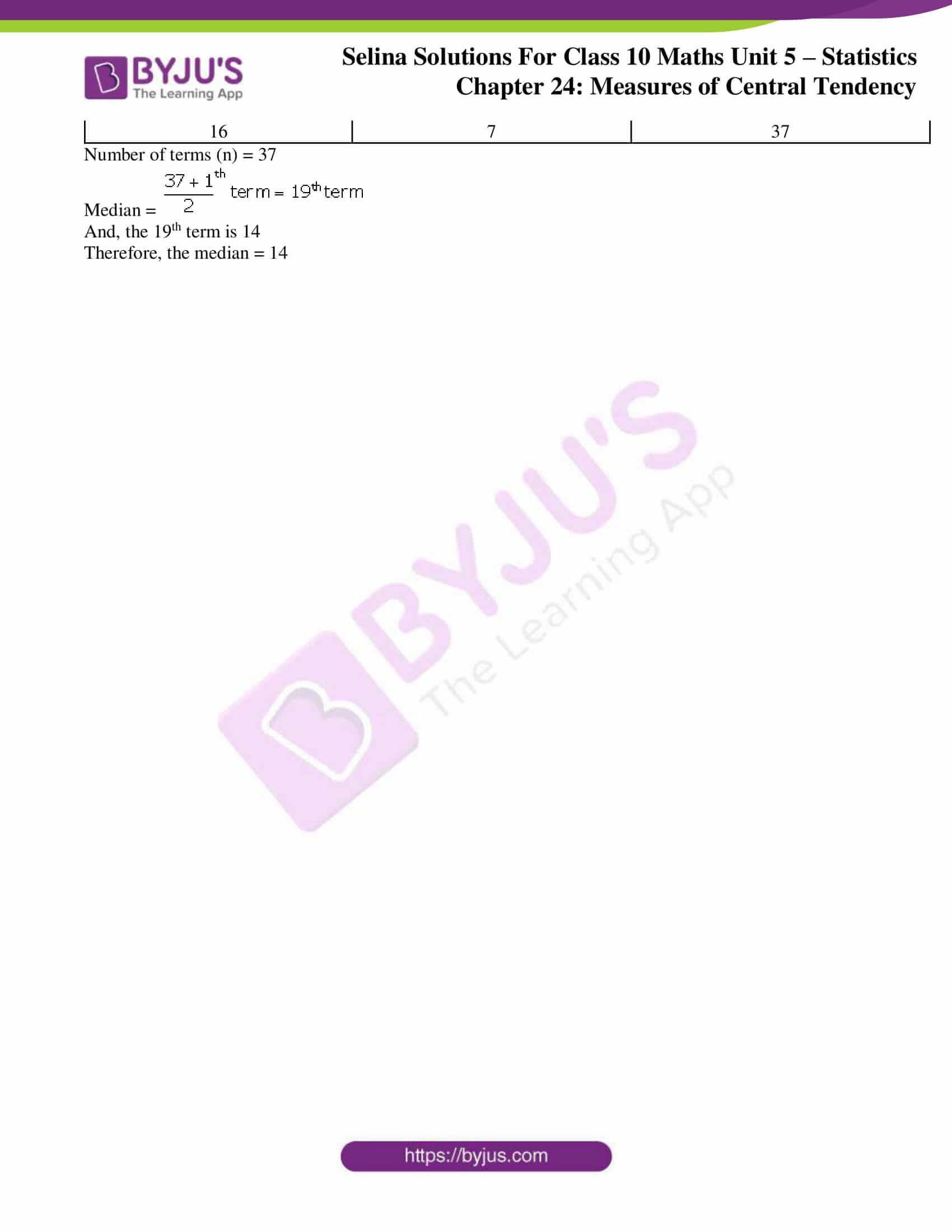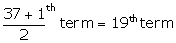# Selina Solutions Concise Maths Class 10 Chapter 24 Measures of Central Tendency Exercise 24(C)

Calculating the median for raw, tabulated and grouped data (both continuous and discontinuous) are the main topics covered under this exercise. In addition, the concept of quartiles are also discussed in the same chapter. Students can access the Selina Solutions for Class 10 Maths for any doubts and clarifications in solving problems of this chapter. The students can also access the solutions of this exercise the Concise Selina Solutions for Class 10 Maths Chapter 24 Measures of Central Tendency Exercise 24(C) in PDF, provided in the link given below.

## Selina Solutions Concise Maths Class 10 Chapter 24 Measures of Central Tendency Exercise 24(C) Download PDF### Access Selina Solutions Concise Maths Class 10 Chapter 24 Measures of Central Tendency Exercise 24(C)

1. A student got the following marks in 9 questions of a question paper.

3, 5, 7, 3, 8, 0, 1, 4 and 6.

Find the median of these marks.

Solution:

Arranging the given data in descending order:

8, 7, 6, 5, 4, 3, 3, 1, 0

Clearly, the middle term is 4 which is the 5th term.

Hence, median = 4

2. The weights (in kg) of 10 students of a class are given below:

21, 28.5, 20.5, 24, 25.5, 22, 27.5, 28, 21 and 24.

Find the median of their weights.

Solution:

Arranging the given data in descending order:

28.5, 28, 27.5, 25.5, 24, 24, 22, 21, 21, 20.5

It’s seen that,

The middle terms are 24 and 24, 5th and 6th terms

Thus,

Median = (24 + 24)/ 2 = 48/2 = 24

3. The marks obtained by 19 students of a class are given below:

27, 36, 22, 31, 25, 26, 33, 24, 37, 32, 29, 28, 36, 35, 27, 26, 32, 35 and 28. Find:

(i) median (ii) lower quartile

(iii) upper quartile (iv) interquartile range

Solution:

Arranging in ascending order:

22, 24, 25, 26, 26, 27, 27, 28, 28, 29, 21, 32, 32, 33, 35, 35, 36, 36, 37

(i) The middle term is 10th term i.e. 29

Hence, median = 29

(ii) Lower quartile(iii) Upper quartile =(iv) Interquartile range = q3 – q1 =35 – 26 = 9

4. From the following data, find:

(i) Median

(ii) Upper quartile

(iii) Inter-quartile range

25, 10, 40, 88, 45, 60, 77, 36, 18, 95, 56, 65, 7, 0, 38 and 83

Solution:

Arranging the given data in ascending order, we have:

0, 7, 10, 18, 25, 36, 38, 40, 45, 56, 60, 65, 77, 83, 88, 95

(i) Median is the mean of 8th and 9th term

Thus, median = (40 + 45)/ 2 = 85/2 = 42.5

(ii) Upper quartile =(iii) Interquartile range is given by,

q1 = 16th/4 term = 18; q3 = 65

Interquartile range = q3 – q1

Thus,

q3 – q1 = 65 – 18 = 47

5. The ages of 37 students in a class are given in the following table:

 Age (in years) 11 12 13 14 15 16 Frequency 2 4 6 10 8 7

Find the median.

Solution:

 Age (in years) Frequency Cumulative Frequency 11 2 2 12 4 6 13 6 12 14 10 22 15 8 30 16 7 37

Number of terms (n) = 37

Median =And, the 19th term is 14

Therefore, the median = 14